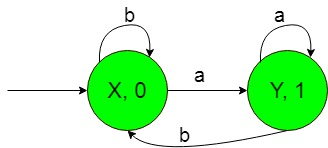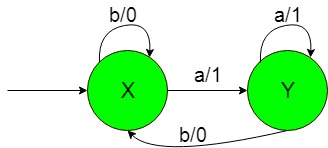TOC | Finite Automata with Output (Set 3)

Prerequisite: Mealy and Moore Machines, Difference between Mealy machine and Moore machine
In this article, we will see some designing of Finite Automata with Output i.e, Moore and Mealy machines.

Problem: Construction of the machines that take set of all string over {a, b} as input and count number of substring ‘a’.
That is here we have,
Ε = {a, b} and
Δ = {0, 1}
where Ε and Δ are the input and output alphabet respectively.
The required Moore machine is constructed below:-Explanation:
In the above diagram, the initial state ‘X’ on getting ‘b’ as the input it remains in the state of itself and print ‘0’ as the output and on getting ‘a’ as the input it transits to a state ‘Y’ and prints ‘1’ as the output. The state ‘Y’ on getting ‘a’ as the input it remains in the state of itself and prints ‘1’ as the output and on getting ‘b’ as the input it comes back to the state ‘X’ and prints ‘0’ as the output.
Thus finally above Moore machine can easily count substring ‘a’ i.e, on getting ‘a’ as the substring it gives ‘1’ as the output thus on counting number of ‘1’ we can count the number of substrings ‘a’.

The required Mealy machine is constructed below:-Explanation:
In the above diagram, the initial state ‘X’ on getting ‘b’ as the input it remains in the state of itself and print ‘0’ as the output and on getting ‘a’ as the input it transits to a state ‘Y’ and prints ‘1’ as the output. The state ‘Y’ on getting ‘a’ as the input it remains in the state of itself and prints ‘1’ as the output and on getting ‘b’ as the input it comes back to the state ‘X’ and prints ‘0’ as the output.
Thus finally above Moore machine can easily count substring ‘a’ i.e, on getting ‘a’ as the substring it gives ‘1’ as the output thus on counting number of ‘1’ we can count the number of substrings ‘a’.My Personal Notes arrow_drop_upCheck out this Author's contributed articles.

If you like GeeksforGeeks and would like to contribute, you can also write an article using contribute.geeksforgeeks.org or mail your article to contribute@geeksforgeeks.org. See your article appearing on the GeeksforGeeks main page and help other Geeks.

Please Improve this article if you find anything incorrect by clicking on the "Improve Article" button below.

Article Tags :

Be the First to upvote.

Please write to us at contribute@geeksforgeeks.org to report any issue with the above content.